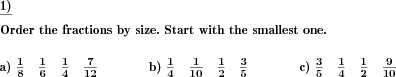Custom math worksheets at your fingertips# Details for problem "Order fractions from least to greatest"

Quickname: 5679

Elementary School, Primary School, Junior High School, Middle School, High School.

## Summary

A series of fractions has to be put in the correct order.

## Example## Description

A number of fractions is presented. These fractions have to be ordered by size, from least to greatest.

All numerators and denominators will be taken from a selectable number range. Note that when converted to like fractions with the same denominator, the common denominator may be much larger.

A simplification may be introduced by specifying that the fractions share the same numerator or denominator.

The number of problems and the number of fractions for a problem can be specified.

Download free printable worksheets for this math problem here. The worksheet contains the problems only, the solution sheet includes the answers. Just click on the respective link.

•Worksheet 1Solution sheet with answers
•Worksheet 2Solution sheet with answers
•Worksheet 3Solution sheet with answers

If you can not see the solution sheets for download, they may be filtered out by an ad blocker that you may have installed. If this is the case, please allow ads for this page and reload the page. The solution sheets will then reappear.

• Do these sample worksheets do not really fit?
• Do you need more math worksheets, with a different level of difficulty?
• Would you like to combine different problems on a worksheet and adjust them to your needs?
• As a teacher, you can put together your own worksheets using the automatically generated math problems provided.
With a free initial credit, you can start creating your own math worksheets in a few minutes.

You can try it for free! Register here, to create custom worksheets now!

## Customization options for this problem

Parameter
Possible values
Number of problems
1, 2, 3, 4, 5, 6, 7, 8, 9, 10
Number range
20, 30, 40, 50, 100, 200, 500, 1000, 10000, 100000
Number of fractions
2, 3, 4, 5
Simplification
None, Same denominator, Same numerator

## Similar problems

Remark
Description
With just two fractions: Fill in the correct relational operator
Insert the correct relational symbol between two fractions